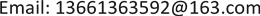1. 引言

2. 二次多项式混沌系统的均匀化处理纸型

b 2 − 4 a c − 2 b ≥ 7

f ( x ) = 1.28 x 2 + 0.56 x − 1.72 , x ∈ [ − 57 32 , 43 32 ] (1)

x ( n + 1 ) = a x 2 ( n ) + b x ( n ) + c (2)

a = 1.28 , b = 0.56 , c = − 1.72.

f ( x ) = 1.28 x 2 + 0.56 x − 1.72 , x ∈ [ − 57 32 , 43 32 ]

g ( x ) = { 2 x , 0 ≤ x ≤ 1 2 2 − 2 x , 1 2 ≤ x ≤ 1

h ( x ) = 25 16 cos π x − 7 32 ,   x ∈ [ 0 , 1 ]

h ∘ g ( x ) = { 25 16 cos 2 π x − 7 32 ,                           0 ≤ x ≤ 1 2 25 16 cos π ( 2 − 2 x ) − 7 32 ,           1 2 ≤ x ≤ 1 = 25 16 cos 2 π x − 7 32 = h (2x)

f ∘ h ( x ) = 32 25 h 2 ( x ) + 14 25 h ( x ) − 43 25 = 32 25 ( h ( x ) + 7 32 ) 2 − 57 32 = 32 25 ( 25 16 cos π x ) 2 − 57 32 = 25 16 cos 2 π x − 7 32 = h (2x)

f ( x ) = 1.28 x 2 + 0.56 x − 1.72 ,   x ∈ [ − 57 32 , 43 32 ]

ρ ( x ) = { 1 π 32 2451 − 448 x − 1024 x 2 , x ∈ [ − 57 32 , 43 32 ] 0 , 其 他

ρ T ( x ) = 1 ,   x ∈ ( 0 , 1 ) .

ρ f ( x ) = ρ T ( h − 1 ( x ) ) | d h − 1 ( x ) d x | = 1 π 32 2451 − 448 x − 1024 x 2

ρ ( x ) = { 1 π 32 2451 − 448 x − 1024 x 2 , x ∈ [ − 57 32 , 43 32 ] 0 , 其 他

Z = 1 π arcsin ( − 16 25 X − 7 50 ) (3)

F Z ( z ) = P ( Z ≤ z ) = P ( 1 π arcsin ( − 16 25 X − 7 50 ) ≤ z ) = ∫ − ∞ − 25 16 sin π z − 7 32 ρ X ( x ) d x (4)

ρ Z ( z ) = { 1 , − 1 2 ≤ z ≤ 1 2 ; 0 ,   其 它 .

{ x ( n + 1 ) = 1.28 x ( n ) 2 + 0.56 x ( n ) − 1.72 z ( n + 1 ) = 1 π arcsin ( − 16 25 x ( n + 1 ) − 7 50 ) (5)

3. 基于均匀化混沌系统构造S-Box3.1. S-Box算法构造

S-Box作为分组密码中的非线性部件，主要起到置换的功能。为了达到更好的置乱效果，增加加密方案的抵抗攻击能力，本文利用混沌系统进行迭代，并基于混沌系统的不同的初始值动态生成 8 × 8 的S-Box，因而，S-Box的密钥由混沌系统的参数，迭代次数，初值等构成。具体的算法步骤为：

1) 将相空间 [ − 0.5 , 0.5 ] 进行n等分，分别为每个小区间标号为 i = 0 , 1 , ⋯ , n ；

2) 取初值 x 0 ，利用混沌系统(5)式迭代n次，得到一个n维混沌序列；

3) 将序列值对应到相空间 [ − 0.5 , 0.5 ] 中的相应小区间，用区间标号 i ( mod 256 ) ，得到一个n维位置序列；

4) 位置序列中前256个不相等的值依次构成S-Box序列。

3.2. S-Box性能分析

，线性运算之和都为128，与理想值相同，满足双射特性，输

S-Box的非线性度为 N s = min       l ∈ L n 0 ≠ u ∈ F 2 m d H ( u ⋅ S ( x ) , l ( x ) ) 。式中： u ⋅ S ( x ) 表示u与 S ( x ) 的点积。表示全体n元线性和仿射函数之集； d H ( x , y ) 表示x和y的汉明距离。函数的非线性度越大，意味着抵抗线性

S-Box的差分概率(DP)用来评价其抵抗差分密码攻击的能力，DP值越小，抵抗能力越强。而线性概率(LP)评价了S-box抵抗线性密码攻击的能力，LP 指标越小，抵抗效果越好。离散混沌S-Box的Lyapunov指数  可以用来衡量双射映射中自变量改变一比特时，映射值变化比特的情况。Lyapunov指数越大，说明自变量能够引起状态值的变成程度越高，意味着系统混沌性越好。本文构造S-Box的DP，LP及其Lyapunov指数结果与文献中S-Box的对比见表3。由对比结果可见，本文S-box的DP值和LP值均小于

S-box sequence sampl
2501744179177633591117161638524718057155
11818219111643751835206187248361605520148
12117539832511711113920410141136145810101
2152435441541102318519719672102213153112232
672810529104663047216242214130157103211252
190385219488125167711222413298221217239195
25416686181129159996038019722422519233
95212249133151377814015015240244402712137
1431316493231185121120212417021812325111
1651894659135444917319925516412822819387108
11322223074145824614914276910616910916196
100237146134132241866911417622138732261728
3122712720989156701884217561686515811919
92486121022023320720312611564521923620213
2007718482253841441622351071786250205179147
2452011522342694208198902383472232296853

Comparison of the nonlinearity of S-boxe
S-Box非线性度均值

Comparison of the DP, LP, Lyapunov exponent of S-boxe
S-BoxDPLPLyapunov

4. 结论